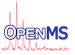OpenMS
Misc functions

Math functions. More...

Collaboration diagram for Misc functions:

## Functions

double ceilDecimal (double x, int decPow)
rounds x up to the next decimal power 10 ^ decPow More...

double roundDecimal (double x, int decPow)
rounds x to the next decimal power 10 ^ decPow More...

double intervalTransformation (double x, double left1, double right1, double left2, double right2)
transforms point x of interval [left1,right1] into interval [left2,right2] More...

double linear2log (double x)
Transforms a number from linear to log10 scale. Avoids negative logarithms by adding 1. More...

double log2linear (double x)
Transforms a number from log10 to to linear scale. Subtracts the 1 added by linear2log(double) More...

bool isOdd (UInt x)
Returns true if the given integer is odd. More...

template<typename T >
round (T x)
Rounds the value. More...

bool approximatelyEqual (double a, double b, double tol)
Returns if a is approximately equal b , allowing a tolerance of tol. More...

template<typename T >
gcd (T a, T b)
Returns the greatest common divisor (gcd) of two numbers by applying the Euclidean algorithm. More...

template<typename T >
gcd (T a, T b, T &u1, T &u2)
Returns the greatest common divisor by applying the extended Euclidean algorithm (Knuth TAoCP vol. 2, p342). Calculates u1, u2 and u3 (which is returned) so that a * u1 + b * u2 = u3 = gcd(a, b, u1, u2) More...

## Detailed Description

Math functions.

These functions are defined in OpenMS/MATH/MISC/MathFunctions.h .

## ◆ approximatelyEqual()

 bool OpenMS::Math::approximatelyEqual ( double a, double b, double tol )
inline

Returns if a is approximately equal b , allowing a tolerance of tol.

## ◆ ceilDecimal()

 double OpenMS::Math::ceilDecimal ( double x, int decPow )
inline

rounds x up to the next decimal power 10 ^ decPow

e.g.: (123.0 , 1)  => 130
(123.0 , 2)  => 200
(0.123 ,-2)  => 0.13 ( 10^-2 = 0.01 )


## ◆ gcd() [1/2]

 T OpenMS::Math::gcd ( T a, T b )

Returns the greatest common divisor (gcd) of two numbers by applying the Euclidean algorithm.

Parameters
 a A number. b A number.
Returns
The greatest common divisor.
gcd(T a, T b, T& a1, T& b1)

References OpenMS::Constants::c.

## ◆ gcd() [2/2]

 T OpenMS::Math::gcd ( T a, T b, T & u1, T & u2 )

Returns the greatest common divisor by applying the extended Euclidean algorithm (Knuth TAoCP vol. 2, p342). Calculates u1, u2 and u3 (which is returned) so that a * u1 + b * u2 = u3 = gcd(a, b, u1, u2)

Parameters
 a A number. b A number. u1 A reference to the number to be returned (see the above formula). u2 A reference to the number to be returned (see the above formula).
Returns
The greatest common divisor.
gcd(T, T)

## ◆ intervalTransformation()

 double OpenMS::Math::intervalTransformation ( double x, double left1, double right1, double left2, double right2 )
inline

transforms point x of interval [left1,right1] into interval [left2,right2]

## ◆ isOdd()

 bool OpenMS::Math::isOdd ( UInt x )
inline

Returns true if the given integer is odd.

Referenced by MorphologicalFilter::filter().

## ◆ linear2log()

 double OpenMS::Math::linear2log ( double x )
inline

Transforms a number from linear to log10 scale. Avoids negative logarithms by adding 1.

Parameters
 x The number to transform

## ◆ log2linear()

 double OpenMS::Math::log2linear ( double x )
inline

Transforms a number from log10 to to linear scale. Subtracts the 1 added by linear2log(double)

Parameters
 x The number to transform

## ◆ round()

 T OpenMS::Math::round ( T x )

Rounds the value.

## ◆ roundDecimal()

 double OpenMS::Math::roundDecimal ( double x, int decPow )
inline

rounds x to the next decimal power 10 ^ decPow

e.g.: (123.0 , 1)  => 120
(123.0 , 2)  => 100